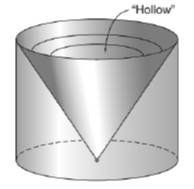Chapter 9.3, Problem 33E### Elementary Geometry for College St...

6th Edition
Daniel C. Alexander + 1 other
ISBN: 9781285195698

#### Solutions

Chapter
Section### Elementary Geometry for College St...

6th Edition
Daniel C. Alexander + 1 other
ISBN: 9781285195698
Textbook Problem
1 views

# A solid is formed by cutting a conical section away from a right circular cylinder. If the radius measures 6 in. and the altitude measures 8 in., what is the volume of the resulting solid?To determine

To find:

The volume of the resulting solid.

Explanation

Given:

A solid is formed by cutting a conical section away from a right circular cylinder. If the radius measures 6 in. and the altitude measures 8 in.

Formula used:

The volume of the right circular cone is V=13πr2h.

The volume of the right circular cylinder is V=πr2h.

Calculation:

Let V1 be the volume of the circular cylinder and let V2 be the volume of the right circular cone.

A solid is formed by cutting a conical section away from a right circular cylinder.

The volume of the resulting solid is V=V1V2.

We have, r=6,h=8.

First let us find V1.

V1=πr2h=π(6)28V1=288π

Now find V2

### Still sussing out bartleby?

Check out a sample textbook solution.

See a sample solution

#### The Solution to Your Study Problems

Bartleby provides explanations to thousands of textbook problems written by our experts, many with advanced degrees!

Get Started

#### In Exercise 11-14, factor the expression. y27y+494

Calculus: An Applied Approach (MindTap Course List)

#### Let f(x) = 1x+1 and g(x) = x2 + 1. Find the rules for (a) f + g, (b) fg (c) f t, and (d) g f.

Applied Calculus for the Managerial, Life, and Social Sciences: A Brief Approach

#### Simplify: 66

Elementary Technical Mathematics

#### Find the length of the curve y=1xt31dt1x4

Single Variable Calculus: Early Transcendentals

#### Solve each proportion. x12=12x+1

College Algebra (MindTap Course List)